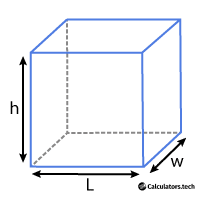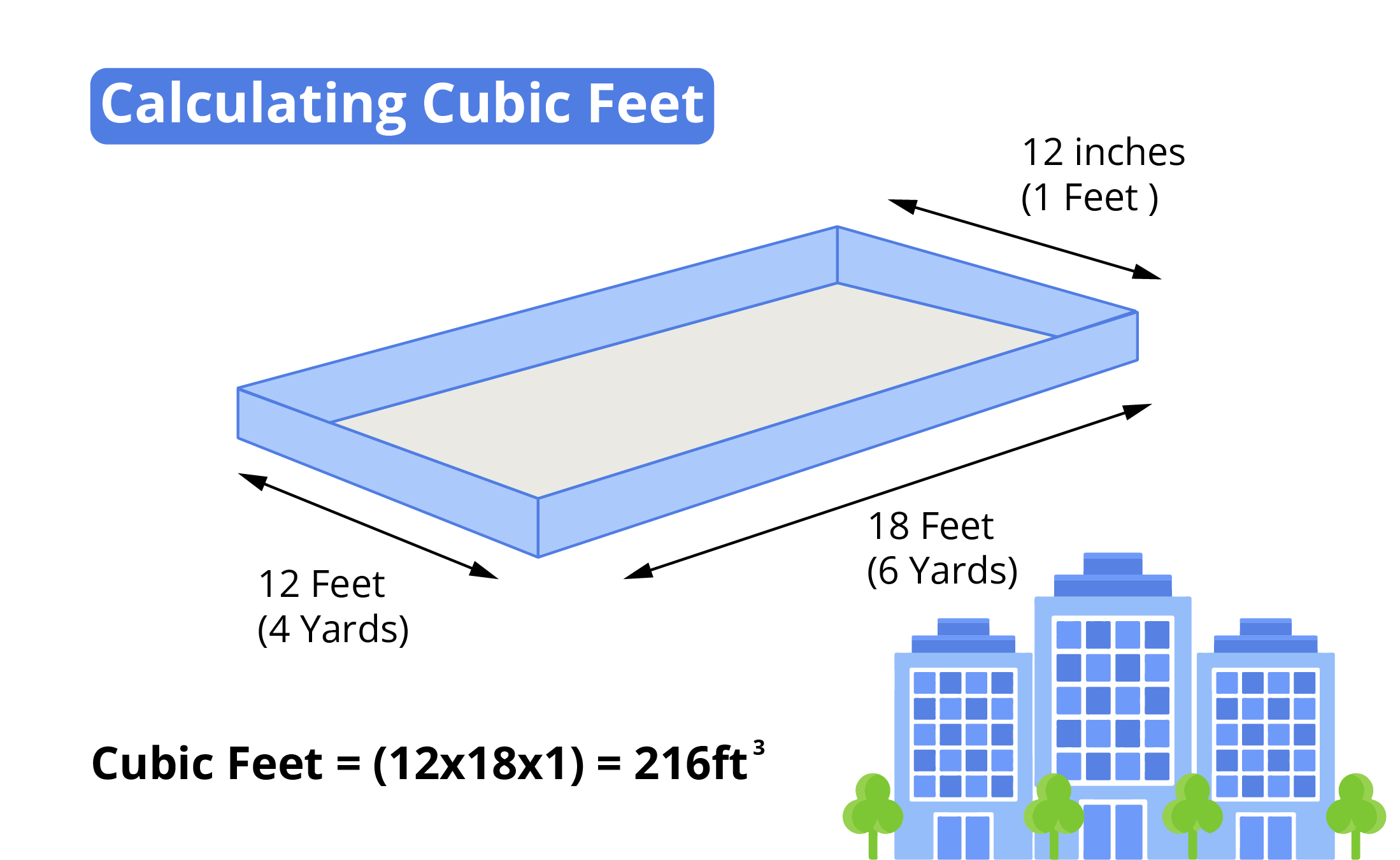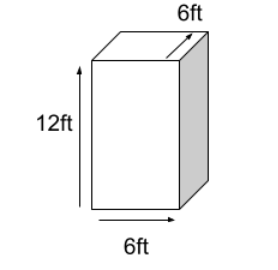# Cubic Feet Calculator

Enter Information

A cubic feet calculator is used to calculate the volume of a cube-shaped space in cubic feet. It will show you the volume in cubic feet with a diagram so that you can grasp the process of calculation.

## How to use the Cubic ft calculator?

To calculate cubic feet using this cubic foot (cuft) calculator, ​​​follow these steps.

1. Enter length, width, and height in the given input boxes.
2. Select the unit of the measurement.
3. Click the "Calculate" button to see the result.

The most common use of this calculator is to convert cubic inches to cubic feet. This calculator can also convert the units such as cft to cbm, cm e.t.c.

If the shape is irregular, split it mentally into regular forms, measure the volume separately for each shape, and add all of them to get the volume in cubic feet.

## What is a Cubic Foot?

A cubic foot is a volume measured in feet for cubical space. It is the volume of a cube equal to one foot with width, length, and height.

In shipping, freight, and landscaping projects, the cubic footage of an object or space is often necessary.## Cubic Feet Formula

Cubic feet = $$\text{Length (feet)} \times \text{Width (feet)} \times \text{Height (feet)}$$

If the shape is irregular, split it mentally into regular forms, measure the volume separately for each shape, and add all of them to get the volume in cubic feet.

## How to Calculate Cubic Feet?

To calculate the volume (cubic feet), measure the given length, width, and height in feet, these terms are in the other units. After that multiply the terms such as:

Length (L) x Width (W) x Height (H)Whenever you want to calculate the capacity of something, use a cubic foot in which three measurements are required such as length, width, and height. For example, if you want to buy a new oven or a window ac. Then measure the place first before placing or fixing that term.

This can be understood with help of an example.

### Example:

Calculate the volume in cubic feet for the given figure.### Solution:

Step 1: Firstly, identify the measurements of different dimensions.

Length = 6 ft

Width = 6 ft

Height = 12 ft

Step 2: Now, multiply all these values to find results.

= L x W x H

= 6 x 6 x 12

= 432 ft3

## Conversion of other units to feet

If you are looking for calculating the volume when you have the measurements in meters, yards, or inches, here are the methods to convert the measurement into similar units such as feet.

### How to measure cubic feet from yards?

If you are looking to find the volume from yards then multiply the given measurements in yards (length, width, and height) then multiply the result by 27. The final result must be in feet. As 1 yard is equal to 3 feet.

Example:

Calculate the capacity of an oven if the length is 12 yards, the width is 6 yards, and the height is 9 yards.

Solution:

Step 1: Multiply the given measurements in yards.

Volume = length x width x height

= 12 yd x 6 yd x 9 yd

= 648 yd3

Step 2: Multiply the result by 27 to convert it into feet.

= 648 x 27 ft3
= 17496 ft3

Alternately

Step 1: Convert the given measurements into feet.

As 1yd = 3ft

Length = 12 yd = 12 x 3 ft = 36 ft

Width = 6 yd = 6 x 3 = 18 ft

Height = 9 yd = 9 x 3 = 27 ft

Step 2: Now multiply the measurements in feet.

Volume = 36ft x 18ft x 27ft

= 17496 ft3

### How to measure cubic feet from inches?

If you are looking to find the volume from inches then multiply the given measurements in inches (length, width, and height), then divide the result by 1728. The final result must be in feet. As 1 inch is equal to 1/12 foot.

Example:

Calculate the capacity of a generator if the length is 20 inches, the width is 60 inches, and the height is 80 inches.

Solution:

Step 1: Multiply the given measurements in inches.

Volume = length x width x height

= 20in x 60in x 80in

= 96000 in3

Step 2: Divide the result by 1728 to convert it into feet.

= 96000 / 1728 ft3

= 55.56 ft3

Alternately

Step 1: Convert the given measurements into feet.

As 1in = 1/12ft

Length = 20in = 20 / 12 ft = 1.67 ft

Width = 60in = 60 / 12 ft = 5 ft

Height = 80in = 80 / 12 ft = 6.67 ft

Step 2: Now multiply the measurements in feet.

Volume = 1.67ft x 5ft x 6.67ft

= 55.56 ft3

### How to measure cubic feet from meters?

If you are looking to find the volume from meters then multiply the given measurements in meters (length, width, and height), then multiply the result by 35.31. The final result must be in feet. As 1 meter is equal to 3.28 feet.

Example:

Calculate the capacity of a dressing table if the length is 5 meters, the width is 6 meters, and the height is 8 meters.

Solution:

Step 1: Multiply the given measurements in meters.

Volume = length x width x height

= 5m x 6m x 8m

= 240 m3

Step 2: Multiply the result by 35.31 to convert it into feet.

= 240 x 35.31 ft3

= 8474.4 ft3

Alternately

Step 1: Convert the given measurements into feet.

As 1m = 3.28ft

Length = 5m = 5 x 3.28 ft = 16.6 ft

Width = 6m = 6 x 3.28 ft = 19.68 ft

Height = 8m = 8 x 3.28 ft = 26.24 ft

Step 2: Now multiply the measurements in feet.

Volume = 16.6ft x 19.68ft x 26.24ft

= 7484.4 ft3

Other Languages
User Ratings
• Total Reviews 0
• Overall Rating 0/5
• Stars
Reviews
No Review Yet